# Can A Function Have Repeating Y Values? (3 Key Ideas)

We know that a function cannot have different y values for a single x value.  However, that still leaves the question of whether different x values can lead to the same y value as output.

So, can a function have repeating y values?  A function can have repeating y values, and a single y value can repeat two or more times. In fact, a y value can repeat infinitely many times for a function. However, a function with repeating y values is not one-to-one, since it will fail the horizontal line test.

Of course, there are lots of functions of both types: those that do not repeat y values (one-to-one functions), and those that do.

Let’s get started.

## Can A Function Have Repeating Y Values?

A function can have repeating y values.  This happens when two inputs (x values) lead to the same output (y value).

This does not violate the definition of a function, which is a relation where each input has only one output.  A repeating y value means we have an output that has more than one corresponding input.

However, a function with repeating y values will not be one-to-one (it will fail the horizontal line test).  As a result, such a function will not have an inverse.

Also remember that an x value cannot be repeated with different y values.  Otherwise, we have a relation that is not a function.

It may help to review the “hierarchy” of relations, functions, and one-to-one functions and the key differences between them.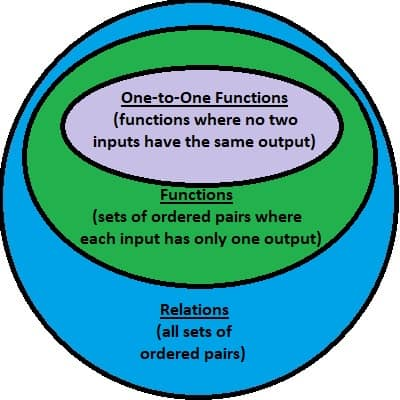A function is a specific type of relation, and a one-to-one function is a specific type of function.

### Relation

A relation is a set of ordered pairs.  A given input can have multiple outputs, meaning that a relation may or may not be a function.

For example, the set {(1, 2), (1, 3)} is not a function, since it has two outputs (2 and 3) for the input 1.  (This set would fail the vertical line test, which tells us that it is not a function.)

A circle is a good example of a relation that is not a function.  The vertical line through the center of a circle intersects the graph twice.

You can see a graph illustrating this below.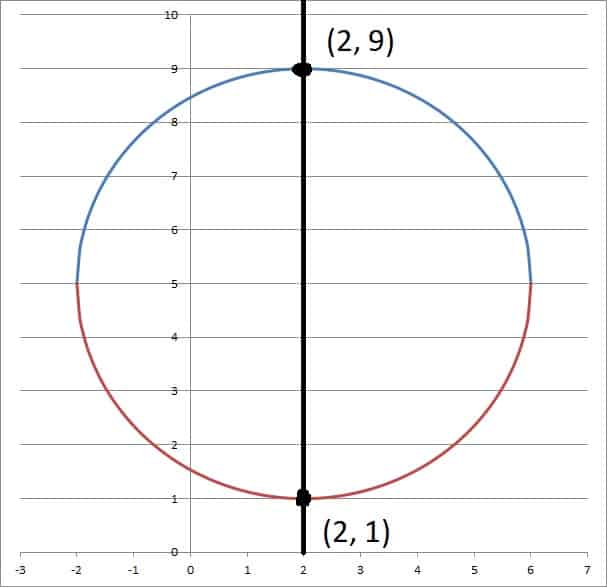A circle is a relation that is not a function. For this circle with center (2, 5) and radius 4, the vertical line x = 2 intersects the graph twice, meaning it fails the vertical line test.

Note: a relation can have repeating y values.

### Function

A function is a relation where each input has only one output.  Two or more different inputs can have the same output (repeating y values).

This means that a function may or may not be one-to-one.  For example, the set {(1, 4), (3, 4)} is a function, since each input has only one output.

However, it is not one-to-one, since two different inputs (1 and 3) lead to the same output (4).  (This set would pass the vertical line test, but it fails the horizontal line test, which tells us that it is not a one-to-one function).

A parabola is a good example of a function that is not one-to-one.  We can find a horizontal line that passes through the parabola twice.

You can see a graph illustrating this below.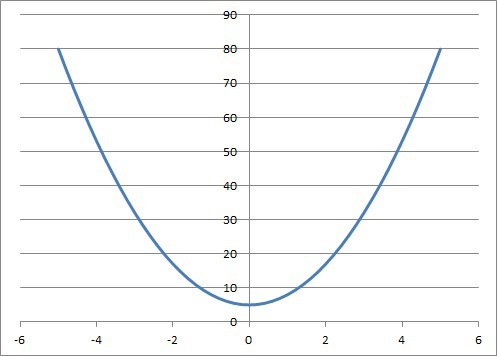A parabola is a function that is not one-to-one. We can see from this graph that the horizontal line y = 10 intersects the parabola twice, so it fails the horizontal line test.

Note: a function can have repeating y values.

### One-To-One Function

A one-to-one function is a function where no two inputs have the same output.  (This set would pass both the vertical line test and the horizontal line test).

A line that is neither horizontal nor vertical is a good example of a one-to-one function.

You can see a graph illustrating this below.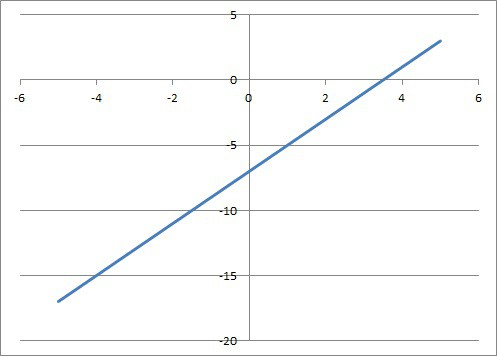This is the graph of the line f(x) = 2x – 7. This is a one-to-one function, since it passes both the vertical line test and the horizontal line test.

Note: a one-to-one function cannot have repeating y values.

## How Many Times Can A Y Value Be Repeated?

A y value can be repeated two or more times for a function.  However, any function with repeated y values is not one-to-one.

A single y value can be repeated up to n times for a polynomial of degree n.

In fact, it is even possible for a y value to repeat infinitely many times for a function! (You can learn about equations with infinitely many solutions here).

Remember that two or more different y values can be repeated for a single function.

Let’s take a look at some examples of functions with repeating y values (note that none of them are one-to-one, since they fail the horizontal line test!)

### Example 1: Function With A Y Value Repeated Twice

Consider the function y = -x2.  This is a parabola that opens downward (it is also an even function).

The value y = -4 is repeated twice, for x = 2 and x = -2.

We can prove this algebraically:

• y = -x2  [parabola equation]
• -4 = -x2  [solving for y = -4]
• 4 = x2
• x = +2, -2  [take positive and negative square roots]

You can see the graph of this parabola (a quadratic function) below.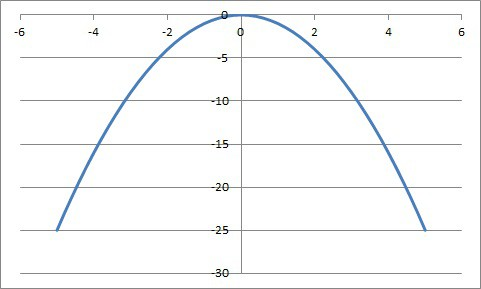The function y = -x2 is a parabola that opens downward. The value y = -4 is repeated twice, at x = 2 and x = -2.

### Example 2: Function With A Y Value Repeated Three Times

Consider the function f(x) = x3 – 6x2 + 11x – 6.  This is a cubic function with three zeroes.

It factors as f(x) = (x – 1)(x – 2)(x – 3).

That means that the value y = 0 is repeated three times, for x = 1, x = 2, and x = 3.

We can prove this algebraically by plugging in x = 1, 2, and 3 to get a y value of zero:

• f(1) = (1)3 – 6(1)2 + 11(1) – 6 = 1 – 6 + 11 – 6 = 0
• f(2) = (2)3 – 6(2)2 + 11(2) – 6 = 8 – 24 + 22 – 6 = 0
• f(3) = (3)3 – 6(3)2 + 11(3) – 6 = 27 – 54 + 33 – 6 = 0

You can see the graph of this cubic function below.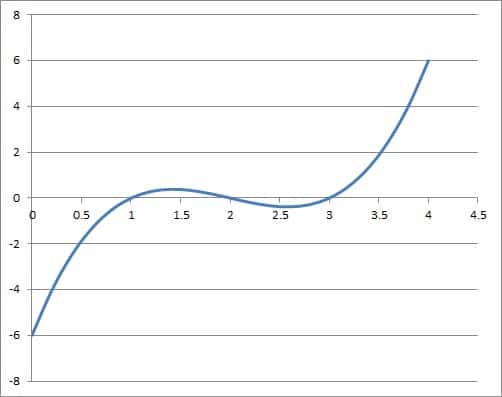The function f(x) = x3 – 6x2 + 11x – 6 is a cubic. The value y = 0 is repeated three times, at x = 1, x = 2, and x = 3.

### Example 3: Function With A Y Value Repeated Four Times

Consider the function f(x) = x4 – 5x2.  This is a quartic function which has the value y = -4 repeated four times.

We can prove this algebraically by setting the function equal to -5 and solving:

• f(x) = -4
• x4 – 5x2 = -4  [definition of f(x)]
• x4 – 5x2 + 4 = 0
• (x2 – 1)(x2 – 4) = 0  [-1 and -4 have a sum of -5 and a product of +4]
• (x + 1)(x – 1)(x + 2)(x – 2) = 0

So the value x = 1, -1, 2, -2 all lead to a y value of -4 for the function f(x) = x4 – 5x2.

You can see the graph of this quartic function below.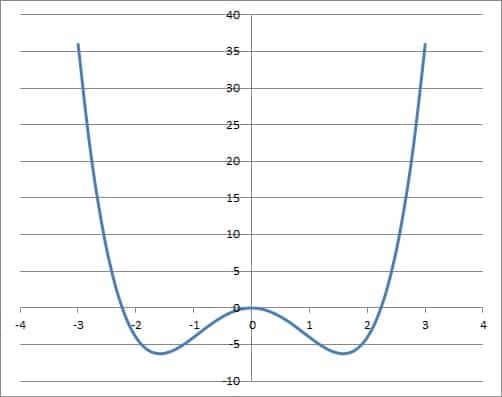The function f(x) = x4 – 5x2 is a quartic. The value y = -4 is repeated four times, for x = -2, x = -1, x = 1, and x = 2.

### Example 4: Function With A Y Value Repeated Infinitely Many Times

Consider the constant function f(x) = -2, which is a horizontal line.  This linear function has the y value -2 repeated infinitely many times.

For any x value you choose, the output (y value) is -2.

You can see the graph of this cubic function below.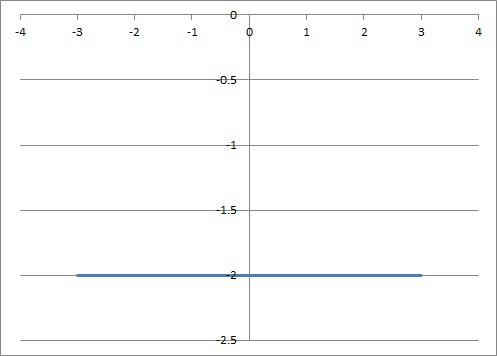The function f(x) = -2 is a horizontal line. The value y = -2 is repeated infinitely many times.

## Conclusion

Now you know that a function can have repeating y values – but a one-to-one function cannot.  You also have some examples to help illustrate the difference between the two types of functions.